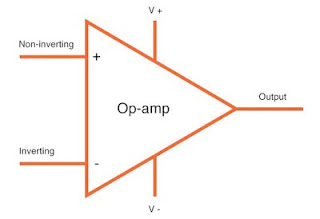# Introduction to Operational Amplifier | Slew Rate

An operational amplifier is a circuit that can perform such mathematical operations as addition, subtraction, integration differentiation. Slew Rate

## Operational Amplifier

An operational amplifier is a circuit that can perform such mathematical operations as addition, subtraction, integration and differentiation. Operational Amplifier, also known as OP-Amp, is basically a voltage amplifying device designed to be used with components like capacitors and resistors, between its input and output terminals.

OP-Amp is a linear devices that are ideal for DC amplification and is used often in signal conditioning, filtering or other mathematical operations. The block diagram of an operational amplifier is shown below. Note that OP- Amp is a multistage amplifier. The three stages are : differential amplifier input stage followed by a high-gain CE (Common-Emitter) amplifier and finally the output stage. The key electronic circuit in an OP-Amp is the differential amplifier.Block diagram of OP-Amp

### An operational amplifier have the following characteristics

1. The input stage of an OP-Amp is a differential amplifier (DA) and the output stage is typically a class B push-pull emitter follower.
2. An operational amplifier has very high input impedance (ideally infinite) and very low output impedance (ideally zero).
3. An operational amplifier has very high open-loop voltage gain ; typically more than 200000.
4. The operational amplifier are almost always operated with negative feedback.

The effect of high input impedance is that the amplifier will draw a very small current (ideally zero) from the signal source. The effect of very low output impedance is that the amplifier will provide a constant output voltage independent of current drawn from the source.

### Schematic Symbol of Operational Amplifier

The basic operational amplifier has five terminal : two terminals for supply voltages +V and -V ; two input terminals (inverting input and non-inverting input) and one output terminal.Schematic Symbol of OP-Amp

## Slew Rate

The slew rate of an OP-AMP is a measure of how fast the output voltage can change and is measured in volts per microsecond (V/µS). Since frequency is a function of time, the slew rate can be used to determine the maximum operating frequency of the OP Amp as follows :

fmax = slew rate / {2πVpk}

Here Vpk is the peak output voltage.